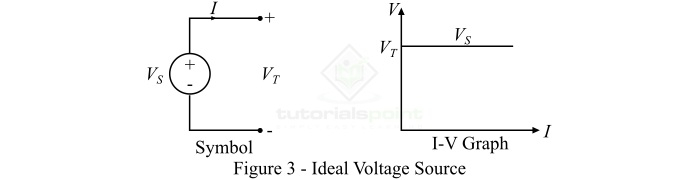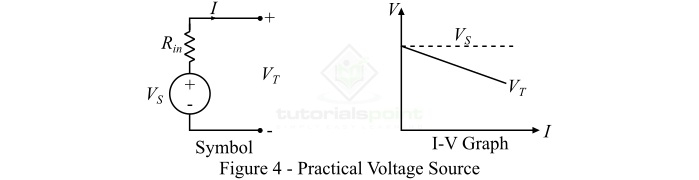# What is a Voltage Source? – Types and Examples

In electrical and electronic circuits, we have to provide necessary for the operation of the circuit. For this purpose, we used energy sources such as voltage sources and current sources. The energy sources are the active circuit elements, i.e. these elements can provide power or power gain in the circuit. In this article, we will discuss what a voltage source is, and different types of voltage sources.

## What is a Voltage Source?

A voltage source is an electric circuit component that is used to create a potential different between two points in an electric circuit. It is basically an active circuit element that provides necessary potential energy in the circuit required to force the electric current through the circuit.

A voltage source is a two terminal device that is connected in a circuit and provide a continuous pressure for the movement of electrons (electric current) in the circuit. The common practical examples of voltage sources are cells, batteries, generators, and other devices that can generate a voltage.

## Types of Voltage Sources

Based on the dependence of voltage, the voltage sources are mainly classified into two types ŌłÆ

• Independent Voltage Sources
• Dependent Voltage Sources

In the following sections of this article, we will discuss in detail about these two types of voltage sources.

## Independent Voltage Sources

The type of voltage source whose voltage does not depend on any other current or voltage in the circuit is known as independent voltage source.

Independent voltage sources are further classified into two types depending on the nature of voltage ŌłÆ

• Direct Voltage Sources
• Alternating Voltage Sources

### Direct Voltage Sources

The type of voltage source which produces a voltage of constant polarity, i.e. which has fixed positive and negative terminals, and these polarities do not change with time is known as a direct voltage source. Based on the magnitude of voltage, direct voltage sources can be either "constant voltage sources" or "time varying voltage sources".

The symbol of direct voltage source is shown in Figure-1.When a direct voltage source is connected in an electric circuit, it always forces an electric current through the circuit that flows from positive terminal to negative terminal, and such a circuit is commonly referred to as a DC circuit. Real examples of direct voltage sources are cells, batteries, solar cells, dc generators, etc.

### Alternating Voltage Sources

A voltage source which produces a voltage whose magnitude changes continuously and the polarity changes periodically is known as alternating voltage source. Therefore, in case of an alternating voltage source, the direction of current through the circuit gets reversed at regular intervals, i.e., the alternating voltage causes the current to flow in one direction for a period of time and after that in the reverse direction for another period of time.

The circuit symbol of alternating voltage source is shown above in Figure-1. The common examples of alternating voltage sources are alternators, inverters, etc.

## Dependent Voltage Sources

The type of voltage source whose output voltage depends on any other voltage or current in the circuit is known as a dependent voltage source. Dependent voltage sources are also known as controlled voltage sources.

The controlled voltage sources are classified into the following two types ŌłÆ

• Voltage Controlled Voltage Source (VCVS)
• Current Controlled Voltage Source (CCVS)

When the output voltage of the voltage source depends upon the voltage in any other part of the electric circuit, then it is known as voltage dependent voltage source (VDVS) or voltage controlled voltage source (VCVS).

On the other hand, when the output voltage of the voltage source depends upon the current in any other part of the circuit, then it is called as current dependent voltage source (CDVS) or current controlled voltage source (CCVS).

Dependent voltage sources are represented by a diamond shaped symbol with polarity marks as shown in Figure-2. The dependent sources are used to model electronic circuits.## Types of Independent Voltage Sources

The independent voltage sources may be also classified into the following two types depending on their internal resistance ŌłÆ

• Ideal Voltage Source
• Practical Voltage Source

## Ideal Voltage Source

A voltage source which has zero internal resistance (or impedance) and can deliver a constant voltage to the circuit to which it connected is known as ideal voltage source. Since an ideal voltage source has zero internal resistance, the voltage across its terminal is equal to the source voltage (or emf). It is because when the current is drawn from an ideal voltage source, there is no voltage drop due to internal resistance. Another important point about the ideal voltage source is that it has 100% efficiency, i.e. there is no power loss in the internal circuit of the ideal voltage source.The circuit symbol and the current/voltage graph of an ideal voltage source is shown in Figure-3. However, ideal voltage sources do not exist in practice, these are just mathematical models of practical voltage sources with no internal losses.

## Practical Voltage Source

A voltage source which has a finite internal resistance and whose terminal voltage drops with the increase in current drawn from it, is known as a practical (or real) voltage source. The circuit symbol and current/voltage graph of a practical voltage source is shown in Figure-4.From the current/ voltage graph of the real voltage source, it is clear that when current drawn from the source increases, the voltage across its terminals decreases. It is because, the increased current increases the voltage drop across the internal resistance. All the voltage sources that we use in actual practical circuits are the practical voltage sources and have certain internal resistance.

## Conclusion

In this article, we discussed in detail about different types of voltage sources. A voltage source provides a constant or variable voltage to an electrical or electronic circuit. The voltage sources act like the excitation system for a circuit that supply required energy for the operation of the circuit.

Based on different parameters, there are several types of voltage sources such as direct voltage sources, alternating voltage sources, dependent voltage sources, ideal voltage sources, practical sources, etc. The common examples of voltage sources are cell, battery, alternator, generator, etc.